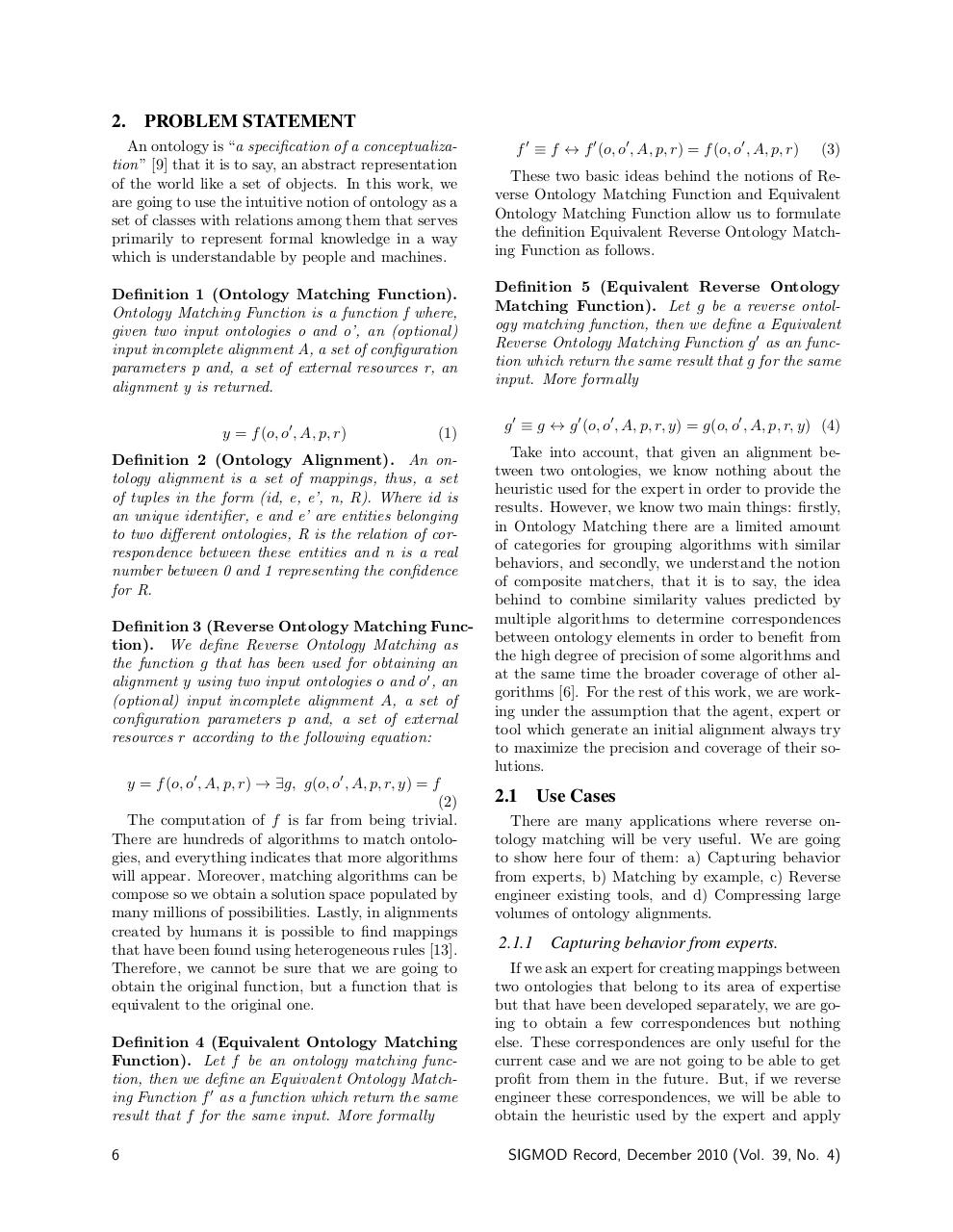# PDF Archive

Easily share your PDF documents with your contacts, on the Web and Social Networks.

## Reverse Ontology Matching.pdfPage 1 2 3 4 5 6 7

#### Text preview

2.

PROBLEM STATEMENT
f 0 ≡ f ↔ f 0 (o, o0 , A, p, r) = f (o, o0 , A, p, r)

An ontology is “a specification of a conceptualization”  that it is to say, an abstract representation
of the world like a set of objects. In this work, we
are going to use the intuitive notion of ontology as a
set of classes with relations among them that serves
primarily to represent formal knowledge in a way
which is understandable by people and machines.

These two basic ideas behind the notions of Reverse Ontology Matching Function and Equivalent
Ontology Matching Function allow us to formulate
the definition Equivalent Reverse Ontology Matching Function as follows.

Definition 1 (Ontology Matching Function).
Ontology Matching Function is a function f where,
given two input ontologies o and o’, an (optional)
input incomplete alignment A, a set of configuration
parameters p and, a set of external resources r, an
alignment y is returned.

Definition 5 (Equivalent Reverse Ontology
Matching Function). Let g be a reverse ontology matching function, then we define a Equivalent
Reverse Ontology Matching Function g 0 as an function which return the same result that g for the same
input. More formally

y = f (o, o0 , A, p, r)

(1)

Definition 2 (Ontology Alignment). An ontology alignment is a set of mappings, thus, a set
of tuples in the form (id, e, e’, n, R). Where id is
an unique identifier, e and e’ are entities belonging
to two different ontologies, R is the relation of correspondence between these entities and n is a real
number between 0 and 1 representing the confidence
for R.
Definition 3 (Reverse Ontology Matching Function). We define Reverse Ontology Matching as
the function g that has been used for obtaining an
alignment y using two input ontologies o and o0 , an
(optional) input incomplete alignment A, a set of
configuration parameters p and, a set of external
resources r according to the following equation:
y = f (o, o0 , A, p, r) → ∃g, g(o, o0 , A, p, r, y) = f
(2)
The computation of f is far from being trivial.
There are hundreds of algorithms to match ontologies, and everything indicates that more algorithms
will appear. Moreover, matching algorithms can be
compose so we obtain a solution space populated by
many millions of possibilities. Lastly, in alignments
created by humans it is possible to find mappings
that have been found using heterogeneous rules .
Therefore, we cannot be sure that we are going to
obtain the original function, but a function that is
equivalent to the original one.
Definition 4 (Equivalent Ontology Matching
Function). Let f be an ontology matching function, then we define an Equivalent Ontology Matching Function f 0 as a function which return the same
result that f for the same input. More formally
6

(3)

g 0 ≡ g ↔ g 0 (o, o0 , A, p, r, y) = g(o, o0 , A, p, r, y) (4)
Take into account, that given an alignment between two ontologies, we know nothing about the
heuristic used for the expert in order to provide the
results. However, we know two main things: firstly,
in Ontology Matching there are a limited amount
of categories for grouping algorithms with similar
behaviors, and secondly, we understand the notion
of composite matchers, that it is to say, the idea
behind to combine similarity values predicted by
multiple algorithms to determine correspondences
between ontology elements in order to benefit from
the high degree of precision of some algorithms and
at the same time the broader coverage of other algorithms . For the rest of this work, we are working under the assumption that the agent, expert or
tool which generate an initial alignment always try
to maximize the precision and coverage of their solutions.

2.1 Use Cases
There are many applications where reverse ontology matching will be very useful. We are going
to show here four of them: a) Capturing behavior
from experts, b) Matching by example, c) Reverse
engineer existing tools, and d) Compressing large
volumes of ontology alignments.

2.1.1

Capturing behavior from experts.

If we ask an expert for creating mappings between
two ontologies that belong to its area of expertise
but that have been developed separately, we are going to obtain a few correspondences but nothing
else. These correspondences are only useful for the
current case and we are not going to be able to get
profit from them in the future. But, if we reverse
engineer these correspondences, we will be able to
obtain the heuristic used by the expert and apply
SIGMOD Record, December 2010 (Vol. 39, No. 4)